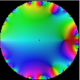# graveolensa

cambridge, ma

http://owen.maresh.info

I'm kind of interested in a lot of bizarre things: extending algebraic topology to take into account strange attractors and whatnot, and categorifying things that probably shouldn't be categorified.
Top Questions

## Visualizing the Partition numbers (suggestions for visualization techniques)

asked Jan 24 '11 at 16:02

## why haven't certain well-researched classes of mathematical object been framed by category theory?

asked Apr 23 '10 at 14:40

## mystery regarding power series of $\frac{1}{\sqrt{1+x^{x}}}$

asked Oct 16 '10 at 8:42

## what is the current state of the art in methods of summing “exotic” series?

asked Oct 15 '10 at 3:04

## What is the combinatoric significance of an integral related to the exponential generating function?

asked Nov 4 '10 at 22:43

## Evaluation of $\int_{0}^{1} \frac{dx}{1+\sqrt[n]{x}}$ for $n\in\mathbb{N}$

asked May 30 '11 at 7:42